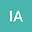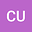loading page

Some new weighted compact embeddings results and existence of weak solutions for eigenvalue Robin problem
••• Ismail Aydin,
• Cihan UNAL
Ismail Aydin
Sinop University
Author ProfileCihan UNAL
Assessment, Selection and Placement Center
Author Profile## Abstract

In this paper, we prove the existence and multiplicity of solutions for the following Robin problem \begin{equation*} \left\{ \begin{array}{cc} -\text{div}\left( a(x)\left\vert \nabla u\right\vert ^{p(x)-2}\nabla u\right) =\lambda b(x)\left\vert u\right\vert ^{q(x)-2}, & x\in \Omega \\ a(x)\left\vert \nabla u\right\vert ^{p(x)-2}\frac{\partial u}{\partial \upsilon }+\beta (x)\left\vert u\right\vert ^{p(x)-2}u=0, & x\in \partial \Omega ,% \end{array}% \right. \end{equation*}% under some appropriate conditions in double weighted variable exponent Sobolev space by applying Mountain Pass Lemma, Ekeland’s variational principle and Fountain Theorem.